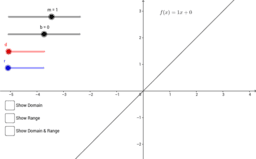# Interactive Exploration of Domain and Range

This GeoGebra book enables students to explore domain and range of a function, y = f(x). As the parameter a changes (using a slider), the corresponding point (a,f(a)) is plotted on the graph. Also, the points (a,0) and (0,f(a)) are plotted with a trace. The traces provide a visual for the domain and range and then students may express in terms of appropriate interval notation.